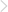返回资讯列表 首页教学基地
2024考研容易陪跑院校：报录比高上岸困难|考研秋季辅导培训班

首都经贸大学

报录比在8:1及以上的专业：工商管理(25.8:1)，法律(非法学)，统计学(10:1)金融硕士(7.82:1)，会计专硕(7.42:1)

报录比在3:1以下的专业：应用经济学(劳动经济学院2.3:1),外国语言文学(2:1)，数量经济学(1.9:1)，电子信息(1.76:1)

西南大学

报录比在8:1以上的专业：金融(14.96:1)，法律(法学)(10.8:1)，教育学原理(15.22:1)，国民经济学-(8.57:1)，课程与教学论(12.24:1)，学前教育学(17.94:1),基础心理学(14.55:1)，学科教学(语文)(19.06:1)，应用心理(23.73:1)，新闻与传播(15.56:1),现代教育技术(12.36:1)，教育史(10.2:1)，教育管理(10.95:1)，会计学(9.7:1)，戏剧与影视学(8.15:1)，文艺学(10.91:1)

报录比在3:1以下的专业：国外马克思主义研究(2.6:1)，学科教学(体育)(1:1)，德语语言文学(3:1)，计算数学(2.25:1)，光学(2.56:1)，化学(2.96:1)，洁净能源科学(1.63:1)，水生生物学(2.63:1)，机械工程(2.64:1)，食品科学(1.81)，机械(1.88:1)，作物遗传育种(2.15:1)，农村发展(1.87:1)，工商管理(1.5:1)，设计学(2.2:1),电子信息(2.65:1)，美学(2:1)，伦理学(1.25:1)，马克思主义哲学(2.51)

福州大学

报录比在8:1以上的专业：企业管理(19.6:1)，心理学(10:1),图书情报(12.921)，金融专硕(9:1)，艺术设计(8.51)，法律(非法学)(7.92:1)

报录比在3:1以下的专业：固体力学(2:1)，，精密机械及测试(1:1)，市政工程(3:1)，资源与环境(2.8:1)，英语语言文学(1.72:1)

西南财经大学

报录比在8:1以上的专业：国民经济学(8.9:1)，企业管理(111)区域经济学(8.78:1)，会计学、法律(非法学)(11:1)，世界经济(11.6:1)，会计学(151)审计(9:1)，新闻与传播(11:1)，行政管理(14:1)应用统计(8.1:1)，金融专硕(8.2:1)，社会工作(8:1)，刑法学(9:1)，英语笔译(8.4:1)，外国语言学及应用语言学(10:1)

报录比在3:1以下的专业：中国近代史基本问题研究(21)，经济哲学(2.4:1)，环境与资源保护法学(3:1)

上海理工大学

报录比在8:1以上的专业：计算机科学与技术(11.7:1)，新闻传播学(13.3:1)，数字出版与传播(18.8:1)，艺术传播(13:1)，英语笔译(8.45:1)，日语笔译(8:1)

报录比在3:1以下的专业：力学(1:1)，系统工程(2.6:1)，化学(2:1)，材料科学与工程(1.4:1)，材料与化工(1.4:1)

河北师范大学

报录比在8:1以上的专业：学科教学(英语)20:1，学科教学(语文)30:1，学科教学(数学)13:1，学科教学(化学)12:1

深圳大学

报录比在8:1以上的专业：教育学(15.8:1)，外国语言文学，学科教学(数学)(21.8;1)，法律(法学)(11:1)，教育管理(15.6:1)，学科教学(语文)(16.7:1)，心理健康教育(12:1)，国际商务(9:1)，应用心理(14.8:1)，心理学(13.34:1)，计算机科学与技术，公共管理(9:1)，法律(非法学)(15:1)，应用统计(9:1)

报录比在3:1以下的专业：材料与化工(1.81)，土木工程(1.1:1)，城乡规划学(3:1)，物理学(1.5:1)

南京大学

报录比在8:1以上的专业：应用心理学，英语笔译，马克思主义哲学，经济法学，中国语言文学。

上海财经大学

报录比在8:1以上的专业：会计学，新闻学，传播学，金融学，社会保障。

东南大学

报录比在8:1以上的专业：建筑学(8.3:1)，风景园林学(23:1)，风景园林(23:1)，工业工程与管理(9:1)，土木工程建造与管理(9.4:1)，管理科学与工程(10:1)，统计学(10:1)，软件工程(10:1)，教育学(20:1)，应用经济学(14.8:1)，金融专硕(9.8:1)，工商管理(9:1)，会计学(9:1)，会计专硕(16:1)，物流工程与管理(12:1)，外国语言学及应用语言学(8:1)，护理专硕(8.11)，心理学(31:1)，应用心理(151)，中国语言文学(81)，中国史(24:1)，旅游管理(111)，艺术学理论(12.2:1)，艺术设计(23:1)，集成电路设计(17:1)

报录比在3:1以下的专业：资源与环境(2.1:1)，能源动力(2.8:1)，光学工程(3:1)，科学与技术教育(2:1)，材料物理与化学(3:1)，材料与化工(2.6:1)，导航制导与控制(31)，内科学(2:1)，汉语国际教育(2:1)，土木水利(苏州联合研究院1.5:1)

上海大学

上海大学

报录比在8:1以上的专业：新闻传播学(28.6:1)，新闻与传播(37.9:1)，法律(非法学)(20.5:1)，戏剧与影视学(27.2:1)，管理科学与工程(18:1)，统计学(9.2:1)

报录比在3:1以下的专业：物理学(3.1:1)，政治经济学(1.2:1)，生物与医药(1.1:1)，微电子学与固体电力学(1:1)、材料与化工(1.7;1)、电子信息(微电子学院1:1)、化学(1:1)、力学(1:1)，微电子学院,机械工程(11)。旅游管理(1.6:1)生物学(1:1)、信号与信息处理(2.3:1)

暨南大学

报录比在8:1以上的专业：区域经济学(18.4:1)，国际商务(18.75:1)，法律法学(10:1)，文艺学(14.75:1)，汉语言文字学(14.2:1)，中国古代文学(22:1)，中国现当代文学(25.3:1)，英语笔译(14:1)，传播学(23.3:1)，计算机应用技术(17.1:1)，管理科学与工程(24.8:1)，国际商务(31.75:1)，行政管理(20.25:1)，材料学(15.3:1)，旅游管理仅接收推免生，放射影像学(11.2:1)，超声医学(13:1)，新闻与传播(12.7:1)，刑法学(18.5:1)

报录比在3:1以下的专业：出版(3.2:1)，材料加工工程一志愿无人上线，应该是进行了校内调剂，材料物理与化学(1.1:1)，环境工程(2.2:1)，资源与环境(2.1:1)，内科学(1.7:1)

郑州大学